# Angle of friction

## Definition

Given two surfaces, the angle of friction (sometimes also termed the angle of repose, though that term has another meaning in a related context) between the two surfaces is defined numerically as: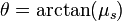$\! \theta = \arctan(\mu_s)$

where$\mu_s$ is the limiting coefficient of static friction.

Here,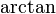$\arctan$ is the arc tangent function, i.e.,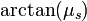$\arctan(\mu_s)$ is the acute angle$\theta$ such that$\tan \theta = \mu_s$.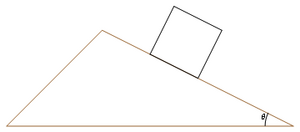The block, if placed gently at rest, starts slipping only if the angle$\theta$ is greater than the angle of friction for the block-incline pair of surfaces.

It can be defined in the following ways:

1. It is the angle$\theta$ that a fixed inclined plane made up of one surface must make with the horizontal so that a body with the second surfaces placed on it just starts slipping. In other words, for any angle smaller than$\theta$, slipping does not begin on its own and for any angle larger than$\theta$, slipping begins on its own. Further information: sliding motion along an inclined plane
2. Consider a block placed on a fixed horizontal plane such that the surfaces of contact are the two desired surfaces. Then,$\theta$ is the angle such that the force needed to pull the block in a particular horizontal direction is minimum if the block is pulled at an angle$\theta$ with that horizontal direction.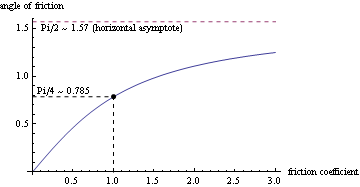The angle of friction in radians, concentrating on the range of typical values of friction coefficient.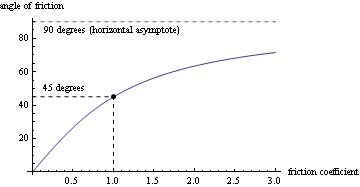The angle of friction in degrees, concentrating on the range of typical values of friction coefficient.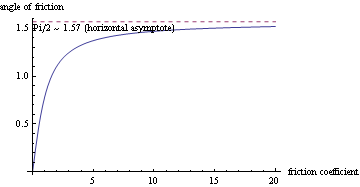The angle of friction in radians, with asymptotic behavior clear.

## Properties

The angle of friction is a strictly increasing function of the limiting coefficient of static friction$\mu_s$, taking the value$0$ for the case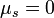$\mu_s = 0$ and approaching the value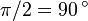$\pi/2 = 90\,^\circ$ as$\mu_s \to \infty$.

## Range of values

First surface Second surface Condition$\mu_s$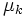$\mu_k$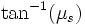$\tan^{-1}(\mu_s)$ (radians)$\tan^{-1}( \mu_s)$ (degrees)$\tan^{-1}(\mu_k)$ (radians)$\tan^{-1}(\mu_k)$ (degrees)
Aluminimum Mild steel Dry and clean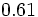$0.61$$0.47$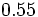$0.55$$31$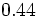$0.44$$25$
Aluminimum Aluminimum Dry and clean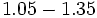$1.05 - 1.35$$1.4$$0.81 - 0.93$$46 - 53$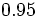$0.95$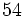$54$
Copper Steel Dry and clean$0.53$$0.36$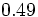$0.49$$28$$0.35$$20$
Copper Copper Dry and clean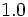$1.0$  ?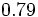$0.79$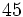$45$  ?  ?
Wood Concrete Dry and clean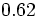$0.62$  ?$0.55$$32$  ?  ?
Wood Metal Dry and clean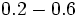$0.2 - 0.6$  ?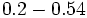$0.2 - 0.54$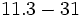$11.3 - 31$  ?  ?
Wood Metal Wet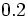$0.2$  ?$0.2$$11.3$
Wood Wood Dry and clean$0.25 - 0.5$  ?$0.24 - 0.46$$14 - 26$  ?  ?
Wood Wood Wet$0.2$  ?$0.2$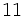$11$  ?  ?
Mild steel Mild steel Dry and clean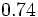$0.74$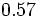$0.57$$0.64$$37$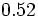$0.52$$30$
Hard steel Hard steel Dry and clean$0.78$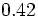$0.42$$0.66$$38$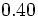$0.40$$23$

For more, see The Engineer's Handbook and Engineering Toolbox.